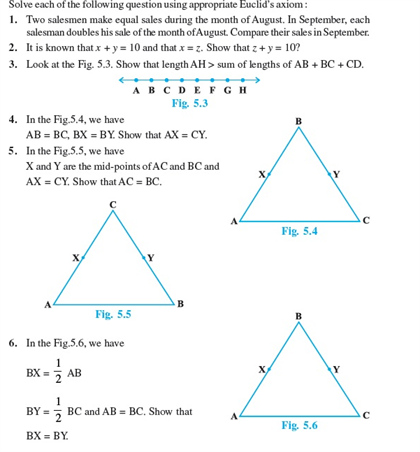# Revision Notes For CBSE Class 9 Math Chapter -5 Introduction to Euclid’s Geometry

The word geometry is derived from the ancient Greek terms which refer to the measurement of the earth. It is a branch of mathematics which mainly deals with the shape, size, area, perimeters, relationships and properties of figures.

Geometry was studied from many ancient civilizations like Greece, Egypt, India, etc. Euclidean geometry is a mathematical system attributed to Alexandrian, a Greek mathematician

Euclidean geometry is the study of plane and solid figures based on five postulates. Listed below are the five basic postulates of Euclidean geometry.

• To draw a straight line from an infinity point.
• To produce a set of straight line.
• To construct a circle with any center and distance.
• To construct a right angle and prove that all right angles are equal to one another.
• To construct the central angles on the same side, less than two right angles on two straight lines.

Check here for the free CBSE Class 9 Maths revision notes for Chapter 5- Introduction to Euclid’s Geometry.